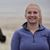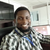# ArcGIS Dashboard Data Expression, "unable to execute Arcade script"

2504
25
04-28-2021 09:14 AMOccasional Contributor III

Hi,

I am very new to Arcade (which you probably be able to tell by my filter expressions below).

I am attempting to create a Data Expression that can be used to power an Indicator widget in a Dashboard. I have built and tested this in the Data Expression builder within my Dashboard and when I click "test" it returns the proper result. I click done, but then see a little triangle with an ! telling me "Unable to execute Arcade script".

Why would I see this message? My Arcade script is below. Any help would be appreciated.

``````var p = 'https://www.arcgis.com';
var itemID_CMpts = 'xxx';
var layerID_CMpts = 0;

var fs1 = FeatureSetByPortalItem(Portal(p), itemID_CMpts, layerID_CMpts, ['Point_Status', 'EQ_File_Number'], false);

var filterCMpts = Filter(fs1, "Point_Status ='Unstable' AND EQ_File_Number ='EQ2015-078' OR Point_Status ='Unstable' AND  EQ_File_Number = 'Eq2013-026' OR Point_Status ='Unstable' AND  EQ_File_Number = 'EQ2018-082' OR Point_Status ='Unstable' AND  EQ_File_Number = 'EQ2018-052' OR Point_Status ='Unstable' AND  EQ_File_Number = 'EQ2001-080X' OR Point_Status ='Unstable' AND  EQ_File_Number = 'EQ2003-021' OR Point_Status ='Unstable' AND  EQ_File_Number = 'EQ1989-015' OR Point_Status ='Unstable' AND  EQ_File_Number = 'EQ2002-023' OR Point_Status ='Unstable' AND  EQ_File_Number = 'EQ2002-045X' OR Point_Status ='Unstable' AND  EQ_File_Number = 'EQ2019-110' OR Point_Status ='Unstable' AND  EQ_File_Number = 'EQ2020-017' OR Point_Status ='Unstable' AND  EQ_File_Number = 'EQ2019-091' OR Point_Status ='Unstable' AND  EQ_File_Number = 'EQ2018-029' OR Point_Status ='Unstable' AND  EQ_File_Number = 'EQ2020-033' OR Point_Status ='Unstable' AND  EQ_File_Number = 'EQ2019-036' OR Point_Status ='Unstable' AND  EQ_File_Number = 'EQ2020-052' OR Point_Status ='Unstable' AND  EQ_File_Number = 'EQ2019-064' OR Point_Status ='Unstable' AND  EQ_File_Number = 'EQ2017-040' OR Point_Status ='Unstable' AND  EQ_File_Number = 'EQ2020-031' OR Point_Status ='Unstable' AND  EQ_File_Number = 'EQ2021-003' OR Point_Status ='Unstable' AND  EQ_File_Number = 'EQ2011-016' OR Point_Status ='Unstable' AND  EQ_File_Number = 'EQ2017-026' OR Point_Status ='Unstable' AND  EQ_File_Number = 'EQ2021-020'");

var itemID_join = 'xxx';
var layerID_join = 0;

var fs2 = FeatureSetByPortalItem(Portal(p), itemID_join, layerID_join, ['Point_Status_revisit', 'EQ_File_Number'], false);

var filterJoin = Filter(fs2, "Point_Status_revisit ='Stable' AND EQ_File_Number ='EQ2015-078' OR Point_Status_revisit ='Stable' AND  EQ_File_Number = 'Eq2013-026' OR Point_Status_revisit ='Stable' AND  EQ_File_Number = 'EQ2018-082' OR Point_Status_revisit ='Stable' AND  EQ_File_Number = 'EQ2018-052' OR Point_Status_revisit ='Stable' AND  EQ_File_Number = 'EQ2001-080X' OR Point_Status_revisit ='Stable' AND  EQ_File_Number = 'EQ2003-021' OR Point_Status_revisit ='Stable' AND  EQ_File_Number = 'EQ1989-015' OR Point_Status_revisit ='Stable' AND  EQ_File_Number = 'EQ2002-023' OR Point_Status_revisit ='Stable' AND  EQ_File_Number = 'EQ2002-045X' OR Point_Status_revisit ='Stable' AND  EQ_File_Number = 'EQ2019-110' OR Point_Status_revisit ='Stable' AND  EQ_File_Number = 'EQ2020-017' OR Point_Status_revisit ='Stable' AND  EQ_File_Number = 'EQ2019-091' OR Point_Status_revisit ='Stable' AND  EQ_File_Number = 'EQ2018-029' OR Point_Status_revisit ='Stable' AND  EQ_File_Number = 'EQ2020-033' OR Point_Status_revisit ='Stable' AND  EQ_File_Number = 'EQ2019-036' OR Point_Status_revisit ='Stable' AND  EQ_File_Number = 'EQ2020-052' OR Point_Status_revisit ='Stable' AND  EQ_File_Number = 'EQ2019-064' OR Point_Status_revisit ='Stable' AND  EQ_File_Number = 'EQ2017-040' OR Point_Status_revisit ='Stable' AND  EQ_File_Number = 'EQ2020-031' OR Point_Status_revisit ='Stable' AND  EQ_File_Number = 'EQ2021-003' OR Point_Status_revisit ='Stable' AND  EQ_File_Number = 'EQ2011-016' OR Point_Status_revisit ='Stable' AND  EQ_File_Number = 'EQ2017-026' OR Point_Status_revisit ='Stable' AND  EQ_File_Number = 'EQ2021-020'");

var CntCMpts = Count(filterCMpts)
var CntJoin = Count(filterJoin)

return CntCMpts - CntJoin``````

Thank you!

Erica
Tags (3)
25 RepliesbyEsri Esteemed Contributor

Hi @erica_tefft ,

I assume that despite the changes it is still not working right? I validated the json that is returned in json lint and it is valid. I have also stored the json in a file and used json 2 feature and it creates a valid featureclass with the features. The only thing that does not come through are the dates. Maybe it is expecting a value (epoch) rather than what it has at this moment. Could you do another test and change the output field type of the date to string. Just to see if that passes. If so we will focus on converting the date to epoch.Occasional Contributor III

That worked! I changed the esriFieldTypeDate to esriFieldTypeString, and it worked perfectly.

Thank you!

EricabyEsri Esteemed Contributor

Hi @erica_tefft , I assume that the date is passing as a string. I provided another above some code to pass the date as epoch which might work.byEsri Esteemed Contributor

Hi @erica_tefft ,

I have a better idea. Can you try this:

``````// settings
var p = 'https://www.arcgis.com';
var itemID_CMpts = 'xxx';
var layerID_CMpts = 0;
var relationshipName = "";

// access first featureset
var fs1 = Filter(FeatureSetByPortalItem(Portal(p), itemID_CMpts, layerID_CMpts, ['Point_Status', 'EQ_File_Number', 'Inspection_Date'], true), "Point_Status ='Unstable' AND EQ_File_Number In ('EQ2015-078', 'Eq2013-026', 'EQ2018-082', 'EQ2018-052', 'EQ2001-080X', 'EQ2003-021', 'EQ1989-015', 'EQ2002-023', 'EQ2002-045X', 'EQ2019-110', 'EQ2020-017', 'EQ2019-091', 'EQ2018-029', 'EQ2020-033', 'EQ2019-036', 'EQ2020-052', 'EQ2019-064', 'EQ2017-040', 'EQ2020-031', 'EQ2021-003', 'EQ2011-016', 'EQ2017-026', 'EQ2021-020')");

// create data schema
var Dict = {
'fields': [
{'name': 'EQNum', 'type': 'esriFieldTypeString'},
{'name': 'InspecDate', 'type': 'esriFieldTypeDate'},
{'name': 'PtStatus', 'type': 'esriFieldTypeString'}],
'geometryType': 'esriGeometryPoint',
"spatialReference": {
"wkid": 102100,
"latestWkid": 3857
},
'features':[]};

// analysis and fill Dict
var i = 0;
for (var f in fs1) {
var fs2 = FeatureSetByRelationshipName(f, relationshipName, ['Point_Status_revisit'], false);
var cnt = Count(fs2);
if (cnt == 0) {
Dict.features[i] = {
'geometry': {
'x': Geometry(f)["X"],
'y': Geometry(f)["Y"]
},
'attributes': {
'EQNum': f["EQ_File_Number"],
'InspecDate': DateDiff(f["Inspection_Date"], Date(1970, 0, 1, 0, 0, 0), "milliseconds"),
'PtStatus': f["Point_Status"]
}
};
i++;
}
}

Console(Text(Dict));

return FeatureSet(Text(Dict));``````Occasional Contributor III

This also works, and provides a nicely formatted time. Thank you for that. I was just digging into this blog to figure out how to format that.

Ericaby
New Contributor

Thank you @XanderBakker  I was cracking my head for days. The DateDiff function solved it for me.